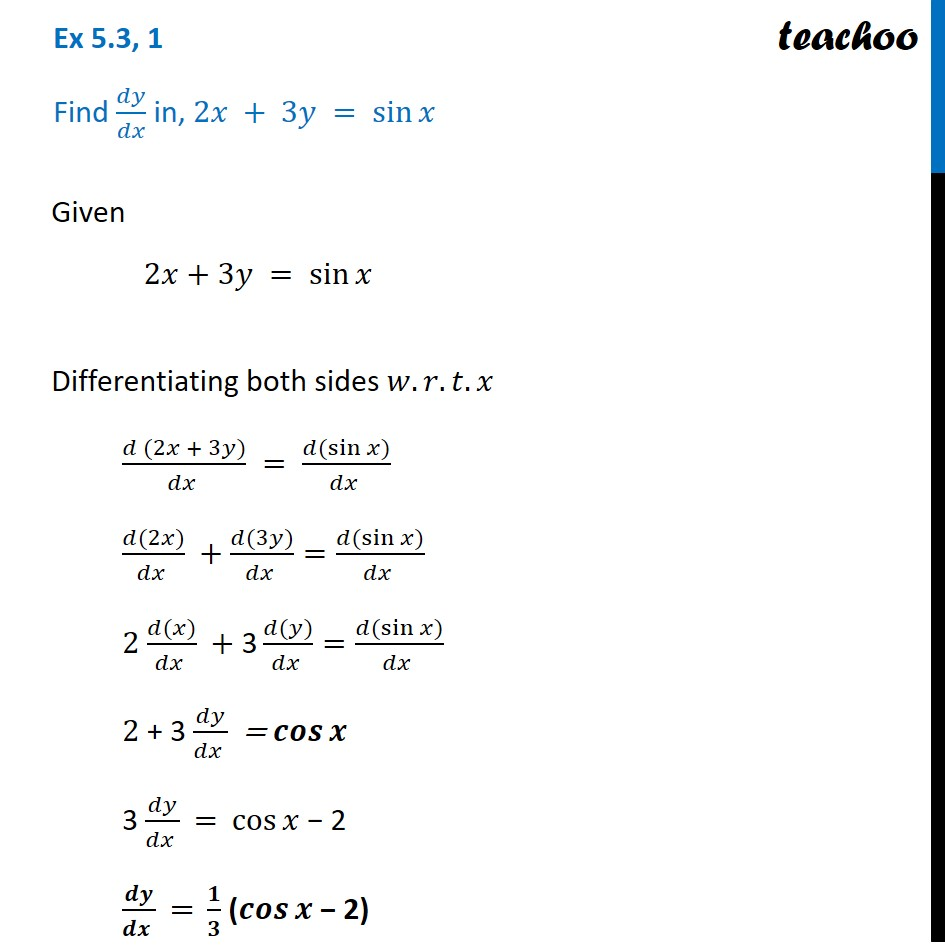Ex 5.3

Chapter 5 Class 12 Continuity and Differentiability
Serial order wiseLearn in your speed, with individual attention - Teachoo Maths 1-on-1 Class

### Transcript

Ex 5.3, 1 Find 𝑑𝑦/𝑑𝑥 in, 2𝑥 + 3𝑦 = sin⁡𝑥 Given 2𝑥+3𝑦 = sin⁡𝑥 Differentiating both sides 𝑤.𝑟.𝑡.𝑥 (𝑑 (2𝑥 + 3𝑦))/(𝑑𝑥 ) = (𝑑(sin⁡〖𝑥)〗)/(𝑑𝑥 ) (𝑑(2𝑥))/(𝑑𝑥 ) +(𝑑(3𝑦))/(𝑑𝑥 )=(𝑑(sin⁡〖𝑥)〗)/(𝑑𝑥 ) 2 (𝑑(𝑥))/(𝑑𝑥 ) +"3" (𝑑(𝑦))/(𝑑𝑥 )=(𝑑(sin⁡〖𝑥)〗)/(𝑑𝑥 ) 2 + 3 𝑑𝑦/(𝑑𝑥 ) = 𝒄𝒐𝒔⁡𝒙 3 𝑑𝑦/(𝑑𝑥 ) = cos⁡𝑥 − 2 𝒅𝒚/(𝒅𝒙 ) = 𝟏/𝟑 (𝒄𝒐𝒔⁡𝒙 − 2)